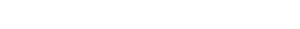Is your handwriting hard to read? Have you ever tried to read something you wrote, but couldn’t because it was so messy? Although we write code by typing, something similar can happen if we aren’t careful.

This lesson will explore the basics of how we can make sure our code is easy to read and understand for ourselves and for others.

Run the example:This code works, but it’s hard to understand for several reasons. Let’s improve it!

There are two variables defined at the top:

`thing1`
and thing2. You can name a variable whatever you want, but it’s best to name it something that tells us what it does.

Change:

`thing1 = …thing2 = …`

to

`user_number = …user_choice = …`

To add a comment in Python, use the # symbol. Python will ignore everything on the line after it, so it won’t mess up your code.

Add comments to the three main if statements in the Rock, Paper, Scissors code to explain what each one does like so: notice the space!

`# If user's number is even and bigger than 5, the robot will choose rock. if … :     … # If user's number is odd and bigger than 5, the robot will choose paper. elif … :     … # Otherwise, the robot will choose scissors. else … :     … `

Consistent  indentation makes code easier to read and can protect it from an

`IndentationError`
.

Correct the bad example code following these rules:

• Anything not in an if statement should start at the “wall.”• Anything inside ONE if statement should start four spaces from the wall.• Anything inside two if statements should start eight spaces from the wall.Look back at the code examples on the previous page.  Notice that when we define a variable, the pattern is

`name = value`
. There is one empty space on each side of the equal sign. Also, the last two examples both have an empty line.

These empty spaces and lines are called whitespace

• Look back at the example code in previous lessons to get a feel for how whitespace can be used for better readability.

### Activity:

Take the Rock, Paper, Scissors robot code you made in the previous lesson and make it ugly. Break the rules about indentation, variable names, and whitespace  that you learned in this lesson. Make sure the code still runs without errors, though!

Ugly Example:

See the code example at the beginning of this lesson.

Good example:

`num = int(input("Enter a number between 1 and 10: ")) user_choice = int(input("Enter 1 for Rock, 2 for Paper, 3 for Scissors: "))# If user's number is less than or equal to 3, Robot will choose rock. if num <= 3:   print("Robot chooses Rock.")# Tell the user the outcome given the robot's choice.   if user_choice == 1:     print("It's a tie!")   elif user_choice == 2:     print("You win!")   else:     print("You lose!")# If user's choice is less than or equal to six, Robot will choose paper. elif num <= 6:   print("Robot chooses Paper.")# Tell the user the outcome given the robot's choice.   if user_choice == 1:     print("You lose!")   elif user_choice == 2:     print("It's a tie!")   else:     print("You win!")`

`# If user's choice is greater than 6, just choose scissors. else:   print("Robot chooses Scissors.")# Tell the user the outcome given the robot's choice.   if user_choice == 1:     print("You win!")   elif user_choice == 2:     print("You lose!")   else:     print("It's a tie.")`